Graph

A set $V$ of vertices and a set $E$ of unordered and ordered pairs of vertices; denoted by $G(V,E)$. An unordered pair of vertices is said to be an edge, while an ordered pair is said to be an arc. A graph containing edges alone is said to be non-oriented or undirected; a graph containing arcs alone is said to be oriented or directed. A pair of vertices can be connected by two or more edges (arcs of the same direction) and such edges (arcs) are then said to be multiple. An arc (or edge) can begin and end at the same vertex, in which case it is known as a loop. (A "graph" is sometimes understood to be a graph without loops or multiple edges; in such a case a graph with multiple edges is said to be a multi-graph, whereas one containing both multiple edges and loops is said to be a pseudo-graph.)

Vertices connected by an edge or a loop are said to be adjacent. Edges with a common vertex are also called adjacent. An edge (arc) and any one of its two vertices are said to be incident. One says that an edge $\{u,v\}$ connects two vertices $u$ and $v$, while an arc $(u,v)$ begins at the vertex $u$ and ends at the vertex $v$. Each graph can be represented in Euclidean space by a set of points, corresponding to the vertices, which are connected by lines, corresponding to the edges (or the arcs) of the graph. In three-dimensional space any graph can be represented in such a way that the lines corresponding to edges (arcs) do not intersect at interior points.

There are various ways of specifying a graph. Let $u_1,\dots,u_n$ be the vertices of a graph $G(V,E)$ and let $e_1,\dots,e_m$ be its edges. The adjacency matrix corresponding to $G$ is the matrix $A=(a_{i,j})$ in which the element $a_{i,j}$ equals the number of edges (arcs) which join the vertices $u_i$ and $u_j$ (go from $u_i$ to $u_j$) and $a_{i,j}=0$ if the corresponding vertices are not adjacent. In the incidence matrix $B=(b_{i,j})$ of $G$ the element $b_{i,j}=1$ if the vertex $u_i$ is incident to the edge $e_j$, and $b_{i,j}=0$ if the vertex $u_i$ and the edge $e_j$ are not incident. A graph can be specified by lists, for example, by specifying pairs of vertices connected by edges (arcs) or by specifying the set of vertices adjacent to each vertex. Two graphs $G(V,E)$ and $H(W,I)$ are called isomorphic if there is a one-to-one correspondence between the sets of vertices $V,W$ and the sets of edges $E,I$ which preserves the incidence relationship (see also Graph isomorphism).

A subgraph $G'(V',E')$ of a graph $G(V,E)$ is defined as a graph with set of vertices $V'\subseteq V$ and set of edges (arcs) $E'\subseteq E$, each one of which is incident with vertices from $V'$ only. A subgraph $G'(V',E')$ is said to be generated or induced by the subset $V'\subseteq V$ if it is a graph with set of vertices $V'$ and ordered set of edges (arcs) $E'$, $E'$ consists of by all edges of $G$ which connect vertices of $V'$. A skeleton subgraph or spanning subgraph $G'(V,E')$ contains all vertices of $G$ and some subset of its edges (arcs) $E'\subseteq E$. A sequence of edges $(u_0,u_1),\dots,(u_{r-1},u_r)$ is called an edge progression or walk connecting the vertices $u_0$ and $u_r$. An edge progression is called a chain or trail if all its edges are different and a simple chain or path if all its vertices are different. A closed (simple) chain is also called a (simple) cycle. A graph is said to be connected if any pair of its vertices is connected by an edge progression. A maximal connected subgraph of a graph $G$ is said to be a connected component. A disconnected graph has at least two connected components (see also Graph, connectivity of a).

The length of an edge progression (chain, simple chain) is equal to the number of edges in the order in which they are traversed. The length of the shortest simple chain connecting two vertices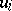and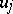in a graphis said to be the distance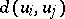betweenand. In a connected undirected graph the distance satisfies the axioms of a metric. The quantityis called the diameter, while a vertex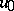for whichassumes its minimum value is called a centre of. A graph can contain more than one centre or no centre at all.

The degree of a vertexof a graph, denoted by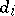, is the number of edges incident with that vertex. If a (loop-free) graphhasvertices andedges, then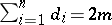. A vertexis said to be isolated ifand terminal or pendant if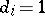. A graph all vertices of which have the same degree (equal to) is said to be regular of degree. A complete graph has no loops and each pair of vertices is connected by exactly one edge. Let a graph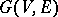be free from loops or multiple edges; then the complementary graph tois the graph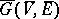in which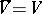and vertices are adjacent in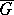only if they are not adjacent in. A graph which is complementary to a complete graph consists of isolated vertices and is known as empty. Many characteristics of a graphand of its complementare related. In a directed graphone defines, for each vertex, the output (or out) and the input (or in) (semi-) degree as the number of arcs issuing from and entering this vertex, respectively. A complete directed graph is known as a tournament. To each graphcan be assigned a number of graphs which are derived from of. Thus, the edge graphofis the graph whose vertices correspond to the edges ofand two vertices are adjacent inif and only if the corresponding edges ofare adjacent. In the total graph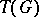ofthe vertices correspond to the elements of, i.e. to vertices and edges, and two vertices inare adjacent if and only if the corresponding elements inare adjacent or incident. Many properties ofcarry over to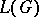and. Many generalizations of the concept of a "graph" are known, including that of a hypergraph and of a network graph.

With the aid of suitable operations it is possible to construct a graph from simpler graphs, to pass from a graph to simpler ones, to subdivide a graph into simpler ones, to pass from one graph to another in a given class of graphs, etc. The most common one-place operations include the removal of an edge (the vertices of the edge are preserved), the addition of an edge between two vertices of a graph, the removal of a vertex together with its incident edges (the graph obtained by removal of a vertexfrom a graphis often denoted by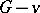), the addition of a vertex (which may be connected by edges with certain vertices of the graph), the contraction of an edge — identification of a pair of adjacent vertices, i.e. removal of a pair of adjacent vertices and addition of a new vertex which is adjacent to those vertices of the graph which were adjacent to at least one of the vertices which have been removed, and subdivision of an edge — removal of an edge and addition of a new vertex which is joined by an edge to each vertex of the edge which has been removed.

Two-place operations over a graph are employed in a number of problems in graph theory. Letand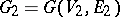be graphs such that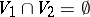and. The union ofand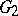is the graphwith set of vertices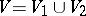and set of edges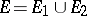. The product of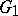andis the graph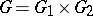whose set of vertices is the Cartesian product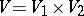, any two of the verticesandbeing adjacent if and only if eitherand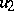is adjacent to, or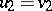and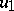is adjacent to. For example, any graph is the union of its connected components; a graph known as the-dimensional unit cube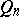can be recursively defined by the product operation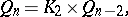where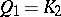is the graph consisting of a pair of vertices connected by one edge. These operations can also be defined for intersecting graphs, in particular for subgraphs of a given graph. The addition moduloof two graphsandis defined as the graphwith set of verticesand set of edges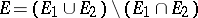. Other many-place operations on graphs are also employed.

For certain classes of graphs it is possible to find simple operations, a repeated application of which makes it possible to pass from any graph in the given class to any other graph in the same class. With the aid of the operation shown in Fig. ait is possible to pass from any graph to any other graph within the class of graphs with the same set of degrees.Figure: g044860a

The operation shown in Fig. bmakes it possible to pass from any triangulation to any other triangulation within the class of planar triangulations (cf. Graph, planar).Figure: g044860b

The description and study of certain classes of graphs also involves operations and sets of graphs making it possible to obtain any graph of a given class. Operations on graphs are also employed to construct graphs with given properties, to calculate numerical characteristics of graphs, etc. (cf. Graph, numerical characteristics of a).

The concept of a "graph" is employed in defining mathematical ideas such as a control system, in certain definitions of an algorithm, of a grammar, etc. The exposition of a number of mathematical theories becomes more easily understood if geometric representations of graphs are employed, e.g. the theory of Markov chains. The concept of a "graph" is widely employed in the formulation and description of various mathematical models in economics, biology, etc.